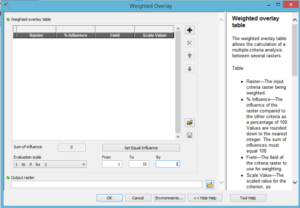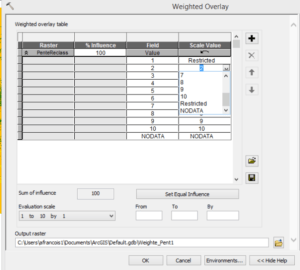# Tutorial: Create an aptitude map with ArcMap, with and without fuzzy criteria (4)

So far we have followed in parallel the two methods of data preparation corresponding to the different criteria for classifying the terrains according to their aptitude to host a new school.

Both methods have resulted in some differences, but it is true that with a little effort, the classical method could be improved and offer equivalent results.

Right now we will deal with the final phase, the aggregation of the four criteria, and here we will face much more significant differences.

Let’s first discuss what is proposed by the classical method, followed by the ESRI Spatial Analyst tutorial.

Merging of the aptitude maps

The final step in the aptitude model is to consolidate reclassified Distance outputs (Aptitude Maps) in relation to the Recreational Facilities, Distance in relation to the existing Schools, Slope and Land Use.

To take into account that some goals are more important than others in the aptitude model, you can weigh the data sets. You will give a higher percentage of influence (weighting) to the more important data sets. If all the data sets are of equal importance, you can give the same weighting to all of them.

In the example, you know, from the examination of the problem, that the first objective is to locate the school near recreational facilities, and the second is to set it far from existing schools. The following percentages of influence will be attributed to the aptitude maps. Values ​​between brackets are the percentages divided by 100 to normalize the values. These are assigned to each aptitude map:

• Distance to recreational facilities: 50%
• Distance to schools: 25%
• Slope: 12.5%
• Land use type: 12.5%

The Distance vs. Recreational Facilities Aptitude Card has an influence of 50% (.5) on the final result, and the Distance from Schools map has an influence of 25% (.25). Slope factors and land use types both have an influence of 12.5% ​​(0.125). As in the assignment of aptitude scales, the weights assignment is a subjective process that relies on the most important goals of your study.

Weighting analysis

What you have just read seems logical, and even intelligent. Do not be offended if you’re a fan of weights, but let’s try to see a little under the surface.

What does it mean to say this mathematical transformation of importance of a criterion using weight value?
And most importantly, remember that the recipient of the result will necessarily be a decision maker to whom we will provide a map to help him make his decision.
We have fully understood that the distance from existing schools and recreation centres criteria are more important for the decision-maker than the type of terrain (slope and occupancy).
Applying these weights, we will (we believe) respect this reasoning of the decision maker.

So, as an example is better than a long speech, let’s take both:

1- A site that is excellent according to the three criteria: distance to schools (value = 10), distance to recreation centres (value = 10) and slope (value = 10), will have as cumulative result for the three criteria (criterion value multiplied by the weight):

0.5 x 10 + 0.25 x 10 + 0.125 x 10 = 8.75

if the land use is the best (agriculture = 10) we will have as final result

8.75 + 1.25 = 10

if the land occupation is the worst (constructed = 3) we will have as final result

8.75 + (0.125 x 3) = 9.125

Do you believe the decision-maker can decide between 9,125 and 10? To the point of realizing that the second choice involves major drawbacks? Do you think that it is obvious at first glance?

So let’s take another even more obvious example:

2- A site that is excellent according to the three criteria: distance to schools (value = 10), distance to recreation centres (value = 10) and land occupation (value = 10) will have the following cumulative result of the three criteria:

0.5 x 10 + 0.25 x 10 + 0.125 x 10 = 8.75

if the slope is 30 ° it will have a value of 7. The final result will be

8.75 + (0.125 x 7) = 9.625

There, one borders perfection.

Therefore a slope of 30 ° corresponds to a slope percentage of 57% which is more than double the feasible limit for a construction project!

How do you think such a proposal will be perceived by the decision maker?

Then, while crossing the criteria we will exclude values ​​from the Slope criterion, with the Restricted option of the weighted overlay. This will prevent proposing sites with slopes in excess of 30%. Then, the only acceptable values ​​of the criterion will be   10, 9 and 8, the others being excluded. And while all the other criteria being constant, between the best slope and the worst, the final result will vary only 0.25!!!

Contribution of slope criterion to the total if slope = 10 -> 10 * 0.125 = 1.25

Contribution of slope criterion to the total if slope = 8-> 8 * 0.125 = 1.00

You can try to change the weights, as much as you want and, you will always find an inadequacy of the results with the human reasoning. Simply because we do not weight criteria in our heads. What mixes up is the fact that we give different importance to criteria together with the fact that we assign a different weight which is not a very good idea.

We will come back later. Now, let’s finish the exercise with Spatial Analyst.

Weighted overlap with Spatial Analyst

Steps   :

1. Open the Weighted Overlay tool. (Spatial Analyst Tools -> Overlay -> Weighted Overlay)2. Type 1, 10, and 1 in the From, To, and By text boxes. The default ranking scale extends from 1 to 9 for each step of 1. A scale from 1   to   10 was used when reclassifying the data sets, before adding input rasters to the Weighted Overlay tool, so you must set the ranking scales from 1   to 10 for each step of 1. This means that you avoid having to update the scale values ​​after adding your input data sets.
3. Click on Apply
4. Add the SlopeReclass layer to the Weighted Overlay tool .
1. Click the Add Raster Line
2. For the input Raster , select   PenteReclass in the drop-down list and leave the input field set to Value .
3. Click OK

The raster is added to the weighted Overlay Table. The Field column displays the values ​​of the SlopeReclass data . The Scale Value column replicates the Field column because the Evaluation Scale has been set to include the range of values ​​for each input raster. At this point, you can change the Scale Values for each class, but for this entry the values ​​have already been properly weighted during the reclassification.
Repeat the previous step for each of the reclassified data sets: LanduseReclass, DistSchoolsReclass, and DistRecSitesReclass .
For the SlopeReclass entry, in the Scale Value column , click the cell that has the value 1.
Click the drop-down arrow, scroll down the view, and then click Restricted.In this way, whatever the values ​​of the other layers, the   resultant value will be 0.

Repeat this for values ​​2 and 3.

For the LandUseReclass layer, set the Scale Value that represents Water and Wetlands (0) to Restricted.

Then, you assign a percentage of influence to each raster, based on the importance (or weighting) of each in the final aptitude card.
In the % Influence column, enter the percentages for each of the input rasters:

DistSchoolsReclass out of 25
DistRecSitesReclass out of 50
SlopeReclass out of 13
LandUseReclass out of 12Click OK to run the tool.

The final result, our aptitude map, appears as follows:The blue areas are the excluded areas (from the weighted overlay Restricted command).

Green areas are the most suitable areas.

In the next article we will perform these operations using the flexible aggregation command, applying fuzzy logic instead of the weights used in Spatial Analyst.